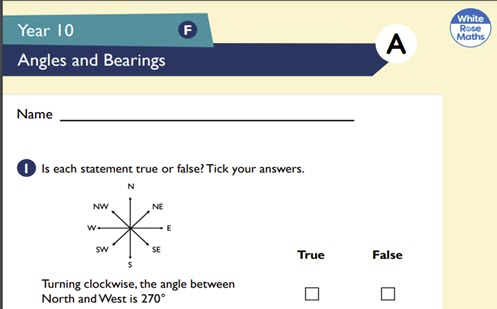# Assessment: angles and bearings

Students apply knowledge of Pythagorean Theorem to problems involving angles of elevation and depression and direction. Each question has an allocated mark.

Year level(s) Year 10
Audience Teacher
Teaching strategies and pedagogical approaches Feedback
Keywords Pythagoras Theorem, Problems

## Curriculum alignment

Strand and focus Space
Topics Pythagoras and trigonometry
AC: Mathematics (V9.0) content descriptions
AC9M10M03

Solve practical problems applying Pythagoras' theorem and trigonometry of right-angled triangles, including problems involving direction and angles of elevation and depression

Numeracy progression Understanding geometric properties (P7)
Understanding units of measurement (P10)